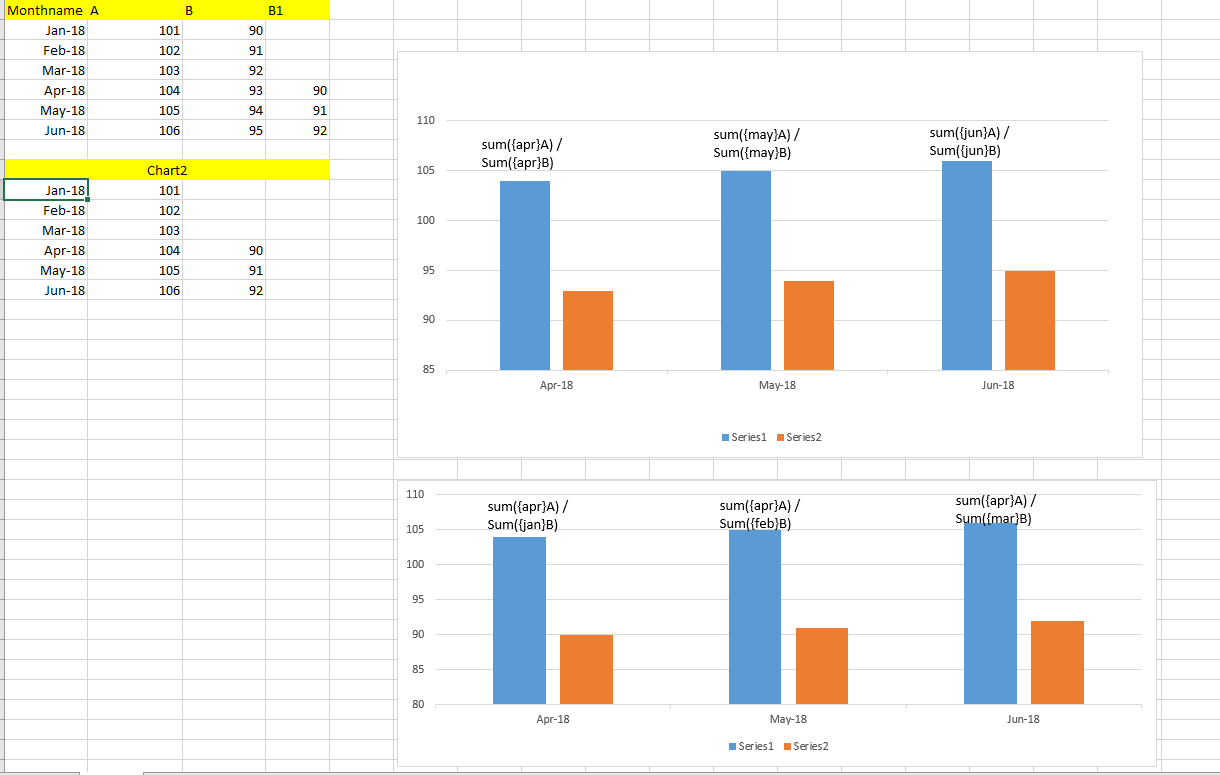# QlikView App Development

Discussion Board for collaboration related to QlikView App Development.

Highlighted
New Contributor III

## How to consider running three months where numerator should be current month and denominator should pick the 3 months previous values in a bar chart?

Hi,

I need to create a bar chart where I need to show the running 3 months as my dimension and in the expression I should consider below logic:

= Sum ( A)

/

Sum ( {<Monthname = {"\$(=MonthName(AddMonths(Monthname,-3)))"} } B)

-> =if(Num(Monthname) >= '\$(=vRunning3Mon)' and Num(Monthname) < '\$(=vRunning3Mon_Max)' ,Monthname)  this is my expression for the Dimension which gives the previous 3 months bars.

-> When I use Monthname = {"\$(=MonthName(AddMonths(Monthname,-3)))"} in my denominator my expression does not get evaluated.

I have also attached a screenshot of desired output

- 1st chart is without any logic i.e., numerator and denominator considers same month. this can be achieved.

- 2nd Chart is with the logic, Numerator will have month from the dimension and my denominator should consider the value 3 months previous to it. Facing issue with this requirement.Tags (2)
7 Replies
Valued Contributor II

## Re: How to consider running three months where numerator should be current month and denominator should pick the 3 months previous values in a bar chart?

Hi,

you have MonthName in dimension,

then the expression sum on MonthName - 3 so you find 0

regards

New Contributor III

## Re: How to consider running three months where numerator should be current month and denominator should pick the 3 months previous values in a bar chart?

Hi Olivier,

even this doesn't work.

RegardsMVP

## Re: How to consider running three months where numerator should be current month and denominator should pick the 3 months previous values in a bar chart?

May be this

Sum(A)/Above(Sum(B), 3)

New Contributor III

## Re: How to consider  running three months where numerator should be current month and denominator should pick the 3 months previous values in a bar chart?

Hi Sunny,

This will not give me the desired output since am already restricting the dimension for only 3 months when we give Above( Sum(B), 3) this will not find any value which is 3 rows above of it.MVP

## Re: How to consider  running three months where numerator should be current month and denominator should pick the 3 months previous values in a bar chart?

Why not? I am not sure what you mean when you say you are restricting the dimension for only 3 months? You are using calculated dimension? If you are... then i suggest not to use that and instead use set analysis

New Contributor III

## Re: How to consider running three months where numerator should be current month and denominator should pick the 3 months previous values in a bar chart?

Yes am using a calculated dimension to get previous 3 months.

I tried this on set analysis, but am having 2 conditions on month itself so I used a calculated dimension and in my set analysis I want to incorporate the logic where for a month the denominator should pick a value which is 3 months back and numerator will have the same month.

ex : if the bar is in may then my exprn should look like

: = Sum( <May> A) / Sum( <Feb> B)

I need help in achieving this.MVP

## Re: How to consider running three months where numerator should be current month and denominator should pick the 3 months previous values in a bar chart?

Would you be able to provide a sample or sample data in an Excel format and based on the sample provided... give us the exact output you are hoping to get?